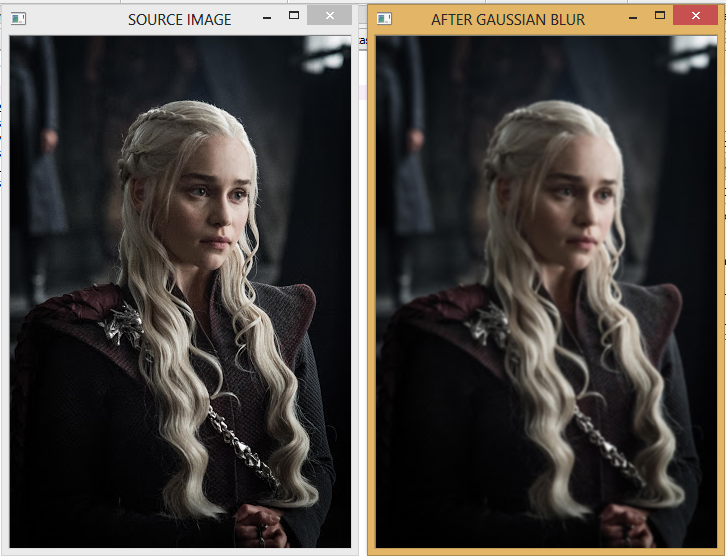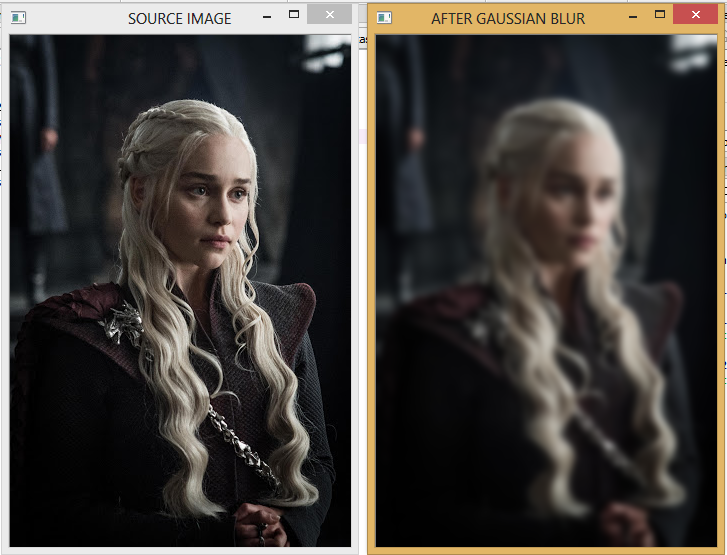# Understanding Gaussian Blur using OpenCV in Python

In this tutorial, we will see:

• What is Gaussian blur?
• How can we apply gaussian blur to our images in Python using OpenCV?

Gaussian Blur is a smoothening technique which is used to reduce noise in an image. Noise in digital images is a random variation of brightness or colour information. This degradation is caused by external sources.

In Gaussian Blur, a gaussian filter is used instead of a box filter. In Python, we can use GaussianBlur() function of the open cv library for this purpose.

To work with open cv, import open cv using:

`import cv2`

### Syntax of GaussianBlur() function in OpenCV – Python

cv2.GaussianBlur(src, ksize, sigmaX[, dst[, sigmaY[, borderType]]])

where,
src: Source image
dst: Output image of same size and type of source image
ksize: Size of Gaussian kernel. It should be odd and positive
sigmaX: Gaussian kernel standard deviation in x direction
sigmaY: Gaussian kernel standard deviation in y direction. If sigmaY=0, it is set equal to sigmaX
borderType: cv2.BORDER_CONSTANT, cv2.BORDER_REPLICATE, cv2.BORDER_REFLECT,      cv2.BORDER_REFLECT_101, cv2.BORDER_TRANSPARENT, cv2.BORDER_REFLECT101, cv2.BORDER_DEFAULT, cv2.BORDER_ISOLATED

### Example 1

```#Gaussian Blur using opencv
import cv2
#showing source image
cv2.imshow("SOURCE IMAGE",img)
#applying gaussian blur
gaus=cv2.GaussianBlur(img,(5,5),0)
#image after gaussian blur
cv2.imshow("AFTER GAUSSIAN BLUR", gaus)
cv2.waitKey(0)
cv2.destroyAllWindows()```

OUTPUT### Example 2

```#Gaussian Blur using open cv
import cv2
#showing source image
cv2.imshow("SOURCE IMAGE",img)
#applying gaussian blur
gaus=cv2.GaussianBlur(img,(21,21),0)
#image after gaussian blur
cv2.imshow("AFTER GAUSSIAN BLUR", gaus)
cv2.waitKey(0)
cv2.destroyAllWindows()```

OUTPUTHope you liked this tutorial!